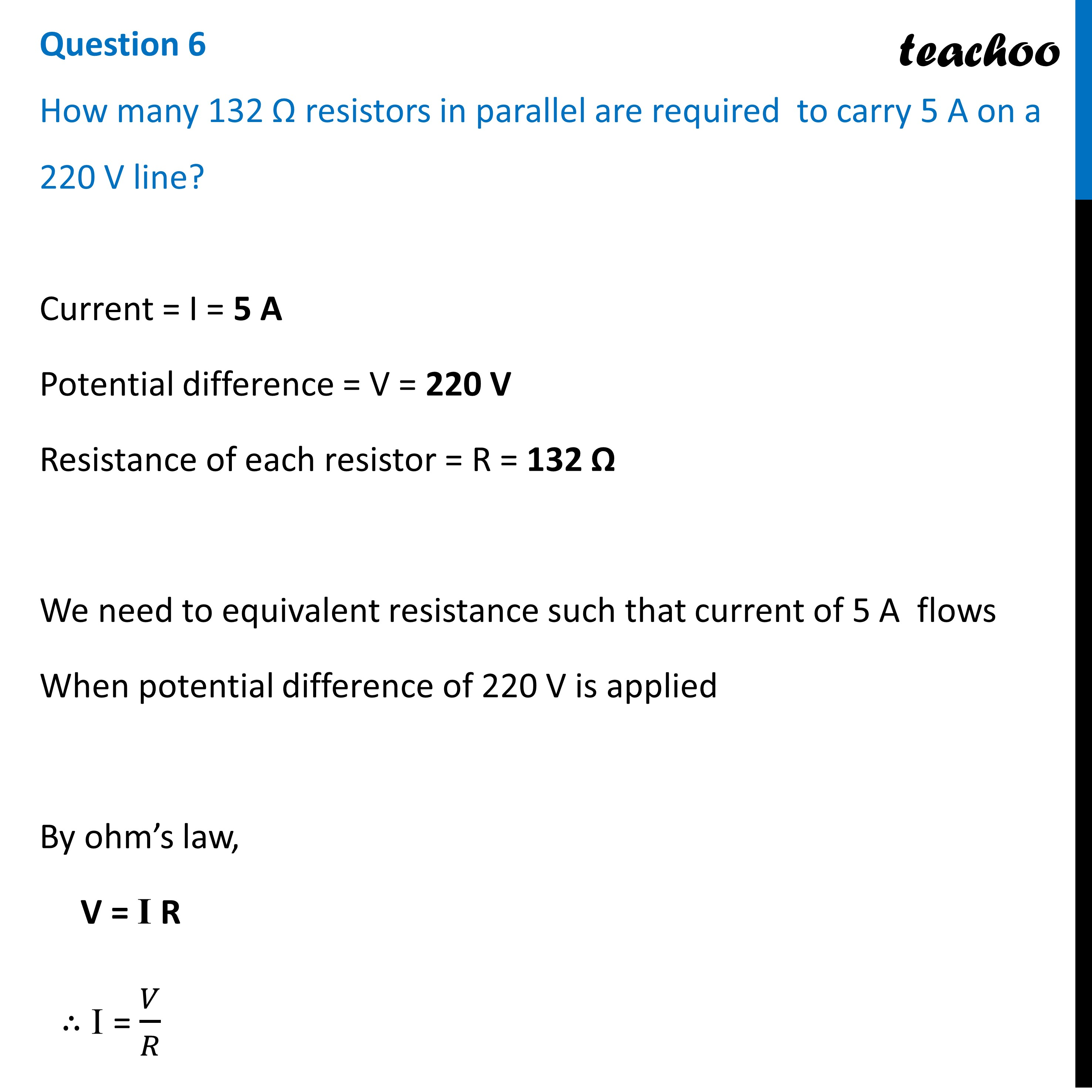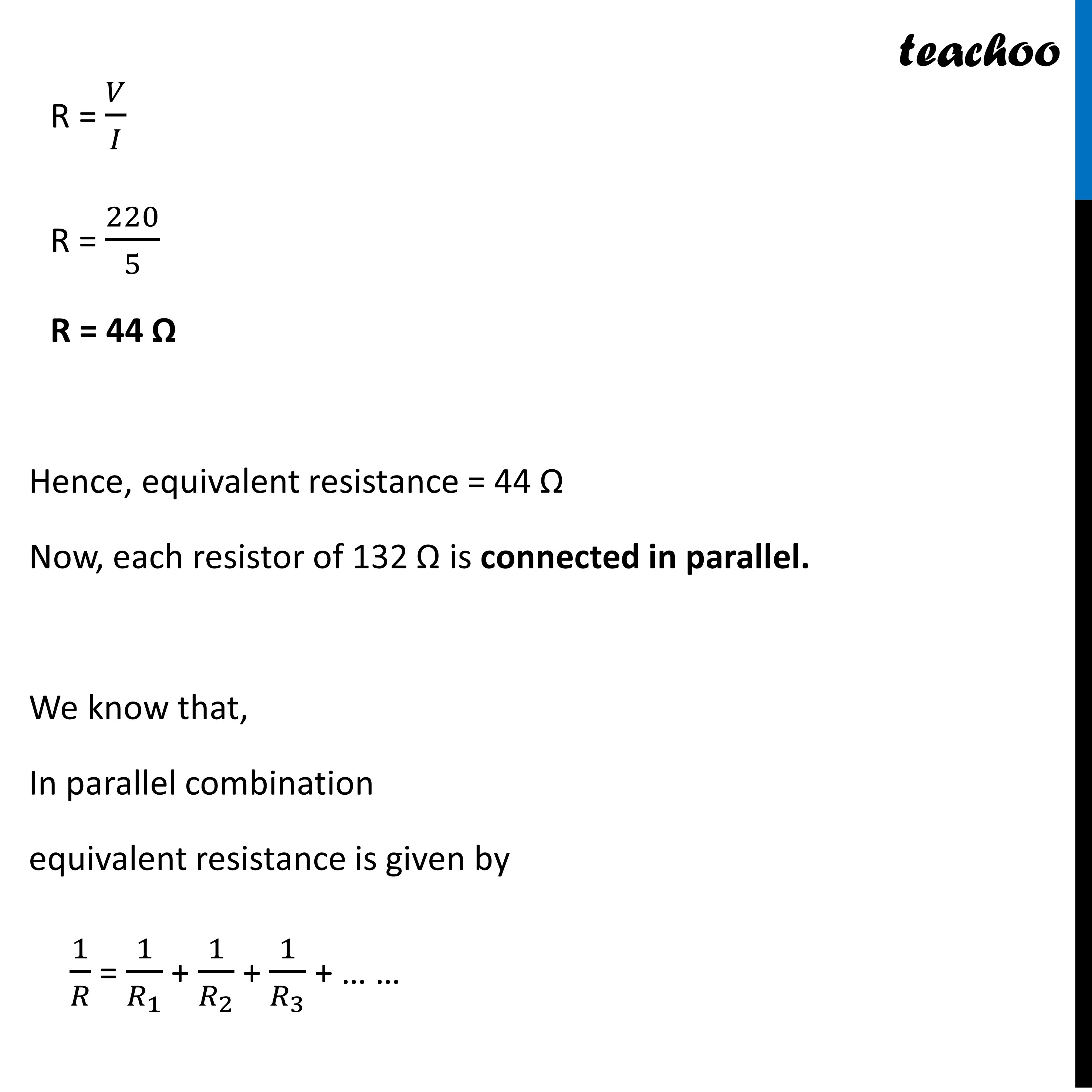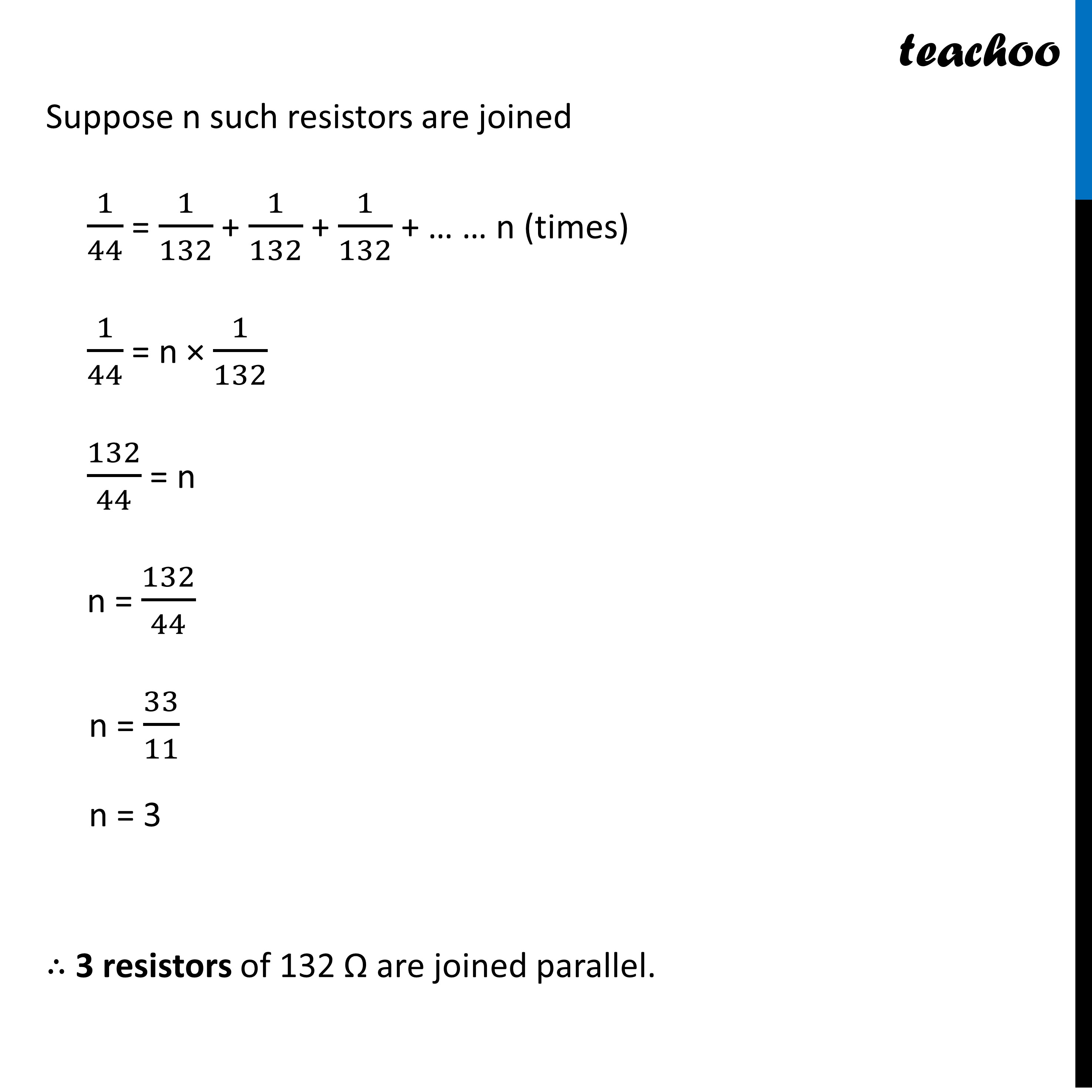Past Year - 2 Mark Questions

Class 10
Chapter 12 Class 10 - Electricity

## How many 132 Ω resistors in parallel are required to carry 5 A on a 220 V line?Learn in your speed, with individual attention - Teachoo Maths 1-on-1 Class

### Transcript

Question 6 How many 132 Ω resistors in parallel are required to carry 5 A on a 220 V line? Current = I = 5 A Potential difference = V = 220 V Resistance of each resistor = R = 132 Ω We need to equivalent resistance such that current of 5 A flows When potential difference of 220 V is applied By ohm’s law, V = I R ∴ I = 𝑉/𝑅R = 𝑉/𝐼 R = 220/5 R = 44 Ω Hence, equivalent resistance = 44 Ω Now, each resistor of 132 Ω is connected in parallel. We know that, In parallel combination equivalent resistance is given by 1/𝑅 = 1/𝑅_1 + 1/𝑅_2 + 1/𝑅_3 + … … Suppose n such resistors are joined 1/44 = 1/132 + 1/132 + 1/132 + … … n (times) 1/44 = n × 1/132 132/44 = n n = 132/44 n = 33/11 n = 3 ∴ 3 resistors of 132 Ω are joined parallel.